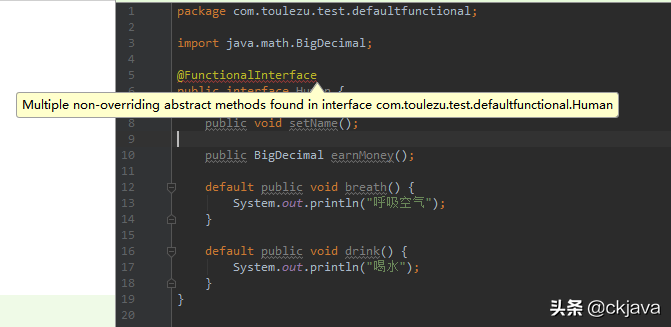# 1 概述

1. Java 8 default 关键字介绍以及使用
2. 对 @FunctionalInterface 注解的理解以及使用

# 2 default 关键字介绍

• default 是在 java8 中引入的关键字，也可称为 Virtual extension methods ——虚拟扩展方法。
• 虚拟扩展方法是指：在接口内部包含了一些默认的方法实现（也就是接口中可以包含方法体，这打破了Java之前版本对接口的语法限制），从而使得接口在进行扩展的时候，不会破坏与接口相关的实现类代码。
• 也就是说：在不修改现有接口实现类的情况下，通过在接口层面增加方法就可以扩展实现类的功能，神奇吧？

# 2.1 为什么要增加 default 关键字

java 8 之前接口中全是抽象方法，好处是面向抽象而不是面向具体编程。

# 2.2 default 关键字的使用

• 定义 Calculate 接口如下
``import java.math.BigDecimal;@FunctionalInterfacepublic interface Calculate<T extends BigDecimal> {    T add(T t1, T t2);    default BigDecimal average(T t1, T t2) {        return add(t1, t2).divide(new BigDecimal(2), 0, BigDecimal.ROUND_HALF_UP);    }}``
• CalculateSub 实现了 Calculate 接口，具体如下
``import java.math.BigDecimal;public class CalculateSub implements Calculate<BigDecimal> {    @Override    public BigDecimal add(BigDecimal t1, BigDecimal t2) {        return t1.add(t2);    }    public static void main(String[] args) {        CalculateSub calculateSub = new CalculateSub();        BigDecimal result = calculateSub.add(BigDecimal.valueOf(2), BigDecimal.valueOf(2));        System.out.println(String.format("result:%s", result)); // result:4        System.out.println(String.format("average:%s", calculateSub.average(BigDecimal.valueOf(4), BigDecimal.valueOf(2)))); // average:3    }}``
• 从上面的例子输出可以看出:
1. 实现类 CalculateSub 也可以使用 Calculate 接口中定义的由 default 关键字修饰的 average 方法
2. 实现类 CalculateSub 中仅重写了 add 方法，这意味着 Calculate 接口中 average 方法（由 default 修饰）可以不用重写了，未来在 Calculate 接口中增加或者减少 default 修饰的方法都不用修改 CalculateSub 类。

# 3.1 @FunctionalInterface 注解的特点

1. 该注解作用于接口类型上
2. 在编译的时候提醒只能有一个抽象方法

@FunctionalInterface 注解在编译的时候如果发现接口中没有或者超过一个抽象方法就会报错：Multiple non-overriding abstract methods found in interface ...，具体如下# 3.2 @FunctionalInterface 注解的使用

``default boolean removeIf(Predicate<? super E> filter) {    Objects.requireNonNull(filter);    boolean removed = false;    final Iterator<E> each = iterator();    while (each.hasNext()) {        if (filter.test(each.next())) {            each.remove();            removed = true;        }    }    return removed;}``

``@FunctionalInterfacepublic interface Predicate<T> {    boolean test(T t);}``

``List<String> dataList = new ArrayList<>();@Beforepublic void init() {    dataList.add("a");    dataList.add("b");    dataList.add("c");}@Testpublic void test_7() {    // 遍历集合，如果发现集合中存在就删除    String str = "a";    for (int i = 0; i < dataList.size(); i++) {        if (dataList.get(i).equals(str)) {            dataList.remove(i);        }    }    // 打印    for (int i = 0; i < dataList.size(); i++) {        System.out.println(dataList.get(i));    }}``

``@Testpublic void test_8() {    // 遍历集合，如果发现集合中存在就删除    String str = "a";    dataList.removeIf(data -> data.equals(str));    // 打印    dataList.forEach(System.out::println);}``

# 3.3 @FunctionalInterface 函数式编程的好处

1. 传入 n 个参数返回个结果
2. 接收 n 个参数，没有返回
3. 接收 n 个参数，返回 boolean 类型
4. 没有参数，返回结果

1. 在遍历集合中进行数据处理，打印，删除，判断，具体的处理可以改成 @FunctionalInterface 接口的
2. if while 中判断，条件可以改成 @FunctionalInterface 接口

``@Testpublic void test_read_file() {    try {        InputStream inputStream = new FileInputStream(new File("D:\\git-workspace\\play\\test-java8-stream\\src\\test\\resources\\test.text"));        BufferedReader bufferedReader = new BufferedReader(new InputStreamReader(inputStream));        String temp = null;        StringBuilder data = new StringBuilder();        while ((temp = bufferedReader.readLine()) != null) {            data.append(temp);        }        JSONObject jsonObject = JSONObject.parseObject(data.toString());        System.out.println(JSONObject.toJSONString(jsonObject, true));    } catch (Exception e) {        e.printStackTrace();    }}``
• 上面这个场景中业务部分是：将文件中的内容存到 StringBuilder 对象中，然后转成 JSONObject 对象，最后打印出来
• 固定的编程套路就是在 while 中通过 bufferedReader 读取文件中的内容

``import java.io.*;import java.util.function.Consumer;public class ReadFile {    public static void processFile(File file, Consumer<String> consumer) {        try {            InputStream inputStream = new FileInputStream(file);            BufferedReader bufferedReader = new BufferedReader(new InputStreamReader(inputStream));            String temp;            while ((temp = bufferedReader.readLine()) != null) {                consumer.accept(temp);            }        } catch (Exception e) {            e.printStackTrace();        }    }}``
• 其中第二个参数是 Consumer 接口对象，通过其中的 accept 方法将 固定的编程套路 和 业务逻辑分离。

``@Testpublic void test_functional() {    File file = new File("D:\\git-workspace\\play\\test-java8-stream\\src\\test\\resources\\test.text");    StringBuilder fileData = new StringBuilder();    ReadFile.processFile(file, data -> fileData.append(data));    JSONObject jsonObject = JSONObject.parseObject(fileData.toString());    System.out.println(JSONObject.toJSONString(jsonObject, true));}``